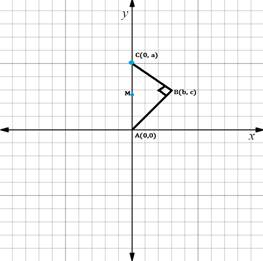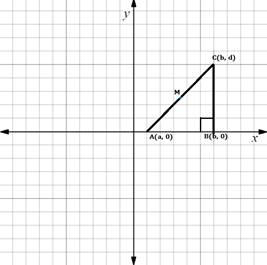Chapter 10.CT, Problem 14CT### Elementary Geometry for College St...

6th Edition
Daniel C. Alexander + 1 other
ISBN: 9781285195698

#### Solutions

Chapter
Section### Elementary Geometry for College St...

6th Edition
Daniel C. Alexander + 1 other
ISBN: 9781285195698
Textbook Problem
1 views

# For providing the theorem “The midpoint of the hypotenuse of a right triangle is equidistant from all three vertices.” which drawing is best?                                 _         a)b)c)To determine

(a)

Which is the best drawing for the given theorem: “The midpoint of the hypotenuse of a right triangle is equidistant from all three vertices.”

Explanation

Given theorem,

The midpoint of the hypotenuse of a right triangle is equidistant from all three vertices

Given drawing,

ABC is the triangle, where

Midpoint of CA is M =0+02,0+

To determine

(b)

Which is the best drawing for the given theorem: “The midpoint of the hypotenuse of a right triangle is equidistant from all three vertices.”

To determine

(c)

Which is the best drawing for the given theorem: “The midpoint of the hypotenuse of a right triangle is equidistant from all three vertices.”

### Still sussing out bartleby?

Check out a sample textbook solution.

See a sample solution

#### The Solution to Your Study Problems

Bartleby provides explanations to thousands of textbook problems written by our experts, many with advanced degrees!

Get Started

#### In Exercises 13-20, sketch a set of coordinate axes and plot each point. 14. (1, 3)

Applied Calculus for the Managerial, Life, and Social Sciences: A Brief Approach

#### For f(x)=exx,f(x)= a) ex b) xex1x2 c) exxexx2 d) xexxx2

Study Guide for Stewart's Single Variable Calculus: Early Transcendentals, 8th

#### 2 1 0 does not exist

Study Guide for Stewart's Multivariable Calculus, 8th

#### List the major sections of an APA-style report in order of appearance.

Research Methods for the Behavioral Sciences (MindTap Course List)